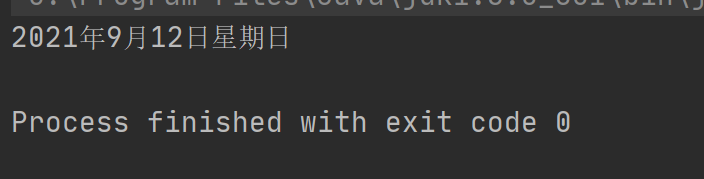• 今天在网上看见一些关于 C#实现的根据年月日计算星期几 的介绍:  算法如下： 基姆拉尔森计算公式 W= (d+2*m+3*(m+1)/5+y+y/4-y/100+y/400) mod 7 在公式中d表示日期中的日数，m表示月份数，y表示年数。 注意：在...函数
• 想了解C/C++根据年月日计算星期几(蔡勒公式篇)的相关内容吗，AresKris在本文为您仔细讲解C/C++计算星期几的相关知识和一些Code实例，欢迎阅读和指正，我们先划重点：c++计算星期几的公式,c语言输入年月日计算星期几,...

想了解C/C++根据年月日计算星期几(蔡勒公式篇)的相关内容吗，AresKris在本文为您仔细讲解C/C++计算星期几的相关知识和一些Code实例，欢迎阅读和指正，我们先划重点：c++计算星期几的公式,c语言输入年月日计算星期几,c语言蔡勒公式，下面大家一起来学习吧。

蔡勒公式  蔡勒(Zeller)公式：是一个计算星期的公式。

随便给一个日期，就能用这个公式推算出是星期几。

蔡勒公式如下：

W = [C/4] - 2C + y + [y/4] + [13 * (M+1) / 5] + d - 1

或者是:

w=y+[y/4]+[c/4]-2c+[26(m+1)/10]+d-1

公式中的符号含义如下：

w：星期； w对7取模得：0-星期日，1-星期一，2-星期二，3-星期三，4-星期四，5-星期五，6-星期六

c：世纪-1(前两位数)

y：年(后两位数)

m：月(m大于等于3，小于等于14，即在蔡勒公式中，某年的1、2月要看作上一年的13、14月来计算，比如2003年1月1日要看作2002年的13月1日来计算)

d：日  [ ]代表取整，即只要整数部分。

下面以中华人民共和国成立100周年纪念日那天(2049年10月1日)来计算是星期几，过程如下：

w=y+[y/4]+[c/4]-2c+[26(m+1)/10]+d-1

=49+[49/4]+[20/4]-2×20+[26×(10+1)/10]+1-1

=49+[12.25]+5-40+[28.6]

=49+12+5-40+28

=54 (除以7余5)

即2049年10月1日(100周年国庆)是星期五。

再比如计算2013年3月7日，过程如下：

w=y+[y/4]+[c/4]-2c+[26(m+1)/10]+d-1

=13+[13/4]+[20/4]-2*20+[26*(3+1)/10]+7-1

=-3 (除以7余4，注意对负数的取模运算！)

示例代码：

int ReturnWeekDay( unsigned int iYear, unsigned int iMonth, unsigned int iDay )

{

int iWeek = 0;

unsigned int y = 0, c = 0, m = 0, d = 0;

if ( iMonth == 1 || iMonth == 2 )

{

c = ( iYear - 1 ) / 100;

y = ( iYear - 1 ) % 100;

m = iMonth + 12;

d = iDay;

}

else

{

c = iYear / 100;

y = iYear % 100;

m = iMonth;

d = iDay;

}

iWeek = y + y / 4 + c / 4 - 2 * c + 26 * ( m + 1 ) / 10 + d - 1; //蔡勒公式

iWeek = iWeek >= 0 ? ( iWeek % 7 ) : ( iWeek % 7 + 7 ); //iWeek为负时取模

if ( iWeek == 0 ) //星期日不作为一周的第一天

{

iWeek = 7;

}

return iWeek;

}

总结

相关文章

展开全文• 介绍了C#根据年月日计算星期几的函数小例子，有需要的朋友可以参考一下
• 通过年月日计算星期的方法：只需要知道三个代码，日期代码+月份代码+年份代码，就可以秒算任意年份任意日期的星期数。
• 输入年月日可以自动计算星期几。Excel表格形式，内部编有计算星期几的程序，只要输入任意年月日就可以计算当天星期几。
• //以1979.12.31为起始日期，星期一，如果是平年下一年的同一天就是星期二，如果是闰年下一年的同一天就是星期三 boolean leap=(year%400==0||(year%4==0&&year%100!=0));//判断是否为闰年 int total=(year-...
int year=2021,month=9,day=12;//以1979.12.31为起始日期，星期一，如果是平年下一年的同一天就是星期二，如果是闰年下一年的同一天就是星期三
boolean leap=(year%400==0||(year%4==0&&year%100!=0));//判断是否为闰年
int total=(year-1980)+(year-1980+3)/4;//year-1980是计算平年增加的天数，（year-1980+3）/4是计算闰年增加的天数，year的起始日期时平年3年后必定是闰年所以加三
for(int i=month-1;i>0;i--){//没有0月，当月的天数不在这里计算所以减1//计算累加的月的天数
switch (i){
case 1: case 3: case 5: case 7: case 8: case 10: case 12: total+=31;break;
case 4: case 6: case 9: case 11: total+=30;break;
case 2:total+=leap?29:28;break;//闰年2月有29天，平年28天
}
}
total+=day;//计算累加的日的天数
int week=1;//因为起始日期为星期一
week=(week+total)%7;
printnb(year+"年"+month+"月"+day+"日"+"星期");
switch (week){
case 1:print(" 1");break;
case 2:print(" 2");break;
case 3:print(" 3");break;
case 4:print(" 4");break;
case 5:print(" 5");break;
case 6:print(" 6");break;
case 0:print("日");break;
}展开全文java
• 你一个年月日，如果要计算那天是星期几了话，我们就可以直接使用基姆拉尔森公式，但是，当月份为1月或者2月时，要让m加上12，同时年份减1，就是当成上一年的13月和14月来算。 if(m==1||m==2) { m+=12; y--; }...

给你一个年月日，如果要计算那天是星期几了话，我们就可以直接使用基姆拉尔森公式，但是，当月份为1月或者2月时，要让m加上12，同时年份减1，就是当成上一年的13月和14月来算。

if(m==1||m==2)
{
m+=12;
y--;
}
x=(d+2*m+3*(m+1)/5+y+y/4-y/100+y/400)%7;

利用此公式求出的余数x，如果x=0，则答案为星期一；x=1，则答案为星期二； x=2，则答案为星期三； x=3，则答案为星期四； x=4，则答案为星期五； x=5，则答案为星期六； x=6，则答案为星期日；。

全部代码如下：

#include<iostream>
#include<cstdio>
#include<cstring>
using namespace std;
int main()
{
int y,m,d,x;
scanf("%d-%d-%d",&y,&m,&d);
if(m==1||m==2)
{
m+=12;
y--;
}
x=(d+2*m+3*(m+1)/5+y+y/4-y/100+y/400)%7;
switch(x)
{
case 0:
cout<<"Monday"<<endl;
break;
case 1:
cout<<"Tuesday"<<endl;
break;
case 2:
cout<<"Wednesday"<<endl;
break;
case 3:
cout<<"Thursday"<<endl;
break;
case 4:
cout<<"Friday"<<endl;
break;
case 5:
cout<<"Saturday"<<endl;
break;
case 6:
cout<<"Sunday"<<endl;
break;
}
return 0;
}

展开全文• 基姆拉尔森计算公式W= (d+2*m+3*(m+1)/5+y+y/4-y/100+y/400) mod 7在公式中d表示日期中的日数，m表示月份数，y表示年数。注意：在公式中有个与其他公式不同的地方：把一月和二月看成是上一年的十三月和十四月，例：...

基姆拉尔森计算公式

W= (d+2*m+3*(m+1)/5+y+y/4-y/100+y/400) mod 7

在公式中d表示日期中的日数，m表示月份数，y表示年数。

注意：在公式中有个与其他公式不同的地方：

把一月和二月看成是上一年的十三月和十四月，例：如果是2004-1-10则换算成：2003-13-10来代入公式计算。

下面是C语言的写的code：

char *CaculateWeekDay(int y,int m,int d){ if(m==1||m==2)  {  m+=12;  y--; }    int week=(d+2*m+3*(m+1)/5+y+y/4-y/100+y/400)%7;    char *weekstr="";    switch(week) {  case 0: weekstr="星期一"; break;  case 1: weekstr="星期二"; break;  case 2: weekstr="星期三"; break;  case 3: weekstr="星期四"; break;  case 4: weekstr="星期五"; break;  case 5: weekstr="星期六"; break;  case 6: weekstr="星期日"; break; } return weekstr;}#include int main(){ int year,month,day; char *str; scanf("%d %d %d",&year,&month,&day); str=CaculateWeekDay(year,month,day);    puts(str); return 0;}

展开全文• t=Integer.parseInt(JOptionPane.showInputDialog("请输入号")); d=n+(n-1)/4-(n-1)/100+(n-1)/400; if(n%4==0) a=28; if(!(n%4==0)) a=29; switch(y){ case'1':x=t-1;break; case'2':x=t+30;break; case'3':x=a+...
• 星期几的判断 输入 一个日期，形式如下： 2018.12.14 输出 周几，数字表示 样例输入 2018.12.14 样例输出 5 基姆拉尔森计算公式。 w=(x+2y+3(y+1)/5+x+x/4+x/100+x/400)%7; x=年份，y=月份，z=天数； 用公年计算的，...
• //传入参数分别为年、月、。 int getWeek(int y, int m, int d) { if(m==1||m==2) { m+=12; y--; } return (d+2*m+3*(m+1)/5+y+y/4-y/100+y/400)%7+1; } int main(void) { char weekDay; ...万年历 week
• 根据年月日计算星期几，例如： 输入：1990 1 1 输出 monday 输入：2013 9 17 输出 tuesday 基姆.拉尔森 给出公式： W=(d+1+2*m+3*(m+1)/5+y+(y>>2)-y/100+y/400)%7;公式
• # include &amp;lt;iostream&amp;gt; # include &amp;lt;string.h&amp;gt; using namespace std; int CaculateWeekDay(int y, int m, int d) { int week = -1;... week = (d+2*m+3...
• 已知具体的年、月、，从而进行相应的星期计算。 核心计算采用蔡基姆拉尔森计算公式 C语言代码如下所示（公式是怎么得出来的，后面有空了继续添加）：   /*引入参数为具体的年月日，例如: Year = 2018; Day...
• #include <... printf("请按格式输入xxxx年xx月xx: "); scanf("%d%d%d",&y,&m,&d); z=(14-m)/12; y0=y-z; x=y0+y0/4-y0/100+y0/400; m0=m+12*z-2; d0=(d+x+31*m0/12)%7; ...c语言 开发语言 后端
• 提示：根据基姆拉尔森日期公式，已知年月日，可以快速的求这一天是星期几。 基姆拉尔森日期公式如下所示: iweek=(d+2∗m+3∗5m+1​+y+4y​−100y​+400y​) mod 7 参数说明： y 为我们输入的年； m ...java 开发语言 后端
• 随便一个日期，就能用这个公式推算星期几。蔡勒公式如下：W = [C/4] - 2C + y + [y/4] + [13 * (M+1) / 5] + d - 1或者是:w=y+[y/4]+[c/4]-2c+[26(m+1)/10]+d-1公式中的符号含义如下：w：星期； w对7取模得：0...
• #include<iostream> using namespace std; int main() { int sum(int,int); int leap(int); int days;//所求第天 int year,month,day;..."输入年月日："; cin>>year>>month>>day...
• 自制程序：输入年月日，以空格隔开，输出当天的星期数。时间范围为公元2000-2600年 代码如下: #include <iostream> using namespace std; int main() { cout<<"输入年月日，以空格隔开（范围公元2000年...
• 基姆拉尔森公式可以用来计算某年某月某日是星期几，下面是使用C#进行演示的代码： using System; using System.Collections.Generic; using System.Linq; using System.Text; using System.Threading.Tasks; ...c# 开发语言 后端
• 随便一个日期，就能用这个公式推算星期几。 蔡勒公式如下： W = [C/4] - 2C + y + [y/4] + [13 * (M+1) / 5] + d - 1 或者是:w=y+[y/4]+[c/4]-2c+[26(m+1)/10]+d-1 公式中的符号含义如下： w：星期； w对...C++ c
• C语言小程序：知任意年月日星期几，蔡勒公式！这个程序有BUG，请需要下载的朋友，下载那个已修正的版本。
• 年月星期计算年月日 C++题目参考资料注意思路c++代码 题目 输入年、月、第周、周几，输出对应的 年、月、。 参考资料 1.https://blog.csdn.net/qq_42403069/article/details/86305578 2....C++ 年月日
• Java实现给定日期计算星期几。通过给定****-**-**的格式来计算星期几
• 大部分日期计算正常， 2014-04-01 （星期2）计算出来的星期不正确。 原因：计算出来的 w可能出现负值， 求负数的余数需要特殊处理，需要按数论的计算方式求余数。网上很多代码存在此问题，少数日期计算不正确。 ...
• C语言源代码，任意年月日，求该天是星期几，修正以前版本中的BUG。菜鸟学编程，希大家多多指教。
• 基姆拉尔森计算公式 W= (d+2*m+3*(m+1)/5+y+y/4-y/100+y/400) mod 7 在公式中d表示日期中的日数，m表示月份数，y表示年数。 注意：在公式中有个与其他公式不同的地方： 把一月和二月看成是上一年的十三月和十四...
• 引入内置模块calendar，输入年、月、，根据weekday(year,month,day)的返回值，输出该日期是星期几。函数weekday()返回0～6分别对应星期一至星期。import calendarfrom calendar import *import datetimey = ......